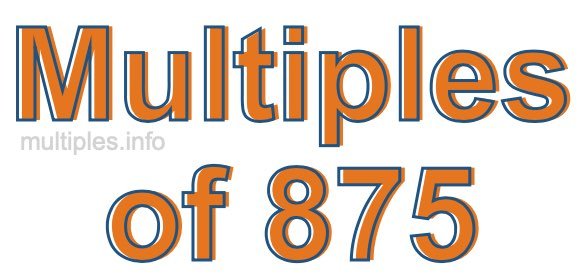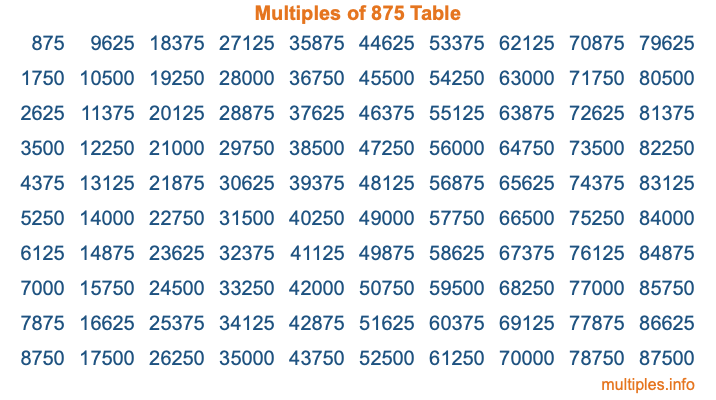Multiples of 875Welcome to the Multiples of 875 page. Here we will first teach you everything you will ever need to know about the multiples of 875, and then give you a study guide summary of everything we taught you to make sure you remember it all. Use this page to look up facts and learn information about the multiples of 875. This page will make you a multiples of eight hundred seventy-five expert!

Definition of Multiples of 875
Multiples of 875 are all the numbers that when divided by 875 equal an integer. Each of the multiples of 875 are called a multiple. A multiple of 875 is created by multiplying 875 by an integer.

Therefore, to create a list of multiples of 875, you start with 1 multiplied by 875, then 2 multiplied by 875, then 3 multiplied by 875, and so on for as long as you want. Thus, the list of the first five multiples of 875 is 875, 1750, 2625, 3500, and 4375. To see a larger list of multiples of 875, see the printable image of Multiples of 875 further down on this page. We also have a category where you can choose any nth multiple of 875.

Multiples of 875 Checker
The Multiples of 875 Checker below checks to see if any number of your choice is a multiple of 875. In other words, it checks to see if there is any number (integer) that when multiplied by 875 will equal your number. To do that, we divide your number by 875. If the the quotient is an integer, then your number is a multiple of 875.

Is  a multiple of 875?

Least Common Multiple of 875 and ...
A Least Common Multiple (LCM) is the lowest multiple that two or more numbers have in common. This is also called the smallest common multiple or lowest common multiple and is useful to know when you are adding our subtracting fractions. Enter one or more numbers below (875 is already entered) to find the LCM.

Check out our LCM Calculator if you need more details about the Least Common Multiple or if you need the LCM for different numbers for adding and subtraction fractions.

nth Multiple of 875
As we stated above, 875 is the first multiple of 875, 1750 is the second multiple of 875, 2625 is the third multiple of 875, and so on. Enter a number below to find the nth multiple of 875.

th multiple of 875

Multiples of 875 vs Factors of 875
875 is a multiple of 875 and a factor of 875, but that is where the similarities end. All postive multiples of 875 are 875 or greater than 875. All positive factors of 875 are 875 or less than 875.

Below is the beginning list of multiples of 875 and the factors of 875 so you can compare:

Multiples of 875: 875, 1750, 2625, 3500, 4375, etc.

Factors of 875: 1, 5, 7, 25, 35, 125, 175, 875

As you can see, the multiples of 875 are all the numbers that you can divide by 875 to get a whole number. The factors of 875, on the other hand, are all the whole numbers that you can multiply by another whole number to get 875.

It's also interesting to note that if a number (x) is a factor of 875, then 875 will also be a multiple of that number (x).

Multiples of 875 vs Divisors of 875
The divisors of 875 are all the integers that 875 can be divided by evenly. Below is a list of the divisors of 875.

Divisors of 875: 1, 5, 7, 25, 35, 125, 175, 875

The interesting thing to note here is that if you take any multiple of 875 and divide it by a divisor of 875, you will see that the quotient is an integer.

Multiples of 875 Table
Below is an image of the first 100 multiples of 875 in a table. The table is in chronological order, column by column. The first column has the first ten multiples of 875, the second column has the next ten multiples of 875, and so on.The Multiples of 875 Table is also referred to as the 875 Times Table or Times Table of 875. You are welcome to print out our table for your studies.

Negative Multiples of 875
Although not often discussed or needed in math, it is worth mentioning that you can make a list of negative multiples of 875 by multiplying 875 by -1, then by -2, then by -3, and so on, to get the following list of negative multiples of 875:

-875, -1750, -2625, -3500, -4375, etc.

Multiples of 875 Summary
Below is a summary of important Multiples of 875 facts that we have discussed on this page. To retain the knowledge on this page, we recommend that you read through the summary and explain to yourself or a study partner why they hold true.

There are an infinite number of multiples of 875.

A multiple of 875 divided by 875 will equal a whole number.

875 divided by a factor of 875 equals a divisor of 875.

The nth multiple of 875 is n times 875.

The largest factor of 875 is equal to the first positive multiple of 875.

875 is a multiple of every factor of 875.

875 is a multiple of 875.

A multiple of 875 divided by a divisor of 875 equals an integer.

875 divided by a divisor of 875 equals a factor of 875.

Any integer times 875 will equal a multiple of 875.

Multiples of a Number
Here you can get the multiples of another number, all with the same attention to detail as we did for multiples of 875 on this page.

Multiples of
Multiples of 876
Did you find our page about multiples of eight hundred seventy-five educational? Do you want more knowledge? Check out the multiples of the next number on our list!